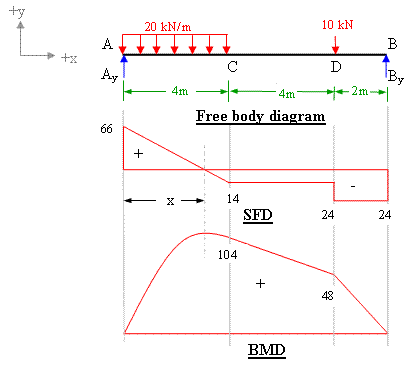# Finding shearing force and moment

## Homework Statement

i couldnt understand why the author take the distance of force 129kN for 4<x<6 and 6<x<8 is x-2 ?

## The Attempt at a Solution

IMO , for 4<x<6 , the distance of force 129kN to the point should be x , where x is the distance from the 129kN to the point of moment , correct me if i am wrong [/B]

#### Attachments

is the author wrong ?

The black dot on the left end of the x axis is the origin (x=0), so the moment arm from x (the point about which the moment is being calculated) to the 192kN force is x-2.

The black dot on the left end of the x axis is the origin (x=0), so the moment arm from x (the point about which the moment is being calculated) to the 192kN force is x-2.
one more thing , why at x = 6 , the shearing force is still 49kN as in the calculation ? why shouldn't it be = 0 ?

why at x = 2 , there are 2 values of shearing forces , namely -40kN (0<x<2) and 89kN (2<x<4) ?

SteamKing
Staff Emeritus
Homework Helper
why at x = 2 , there are 2 values of shearing forces , namely -40kN (0<x<2) and 89kN (2<x<4) ?
Because the reaction RA is located at x = 2. As you move along the beam from left to right, the shear force has a sudden jump from V = -40 kN to V = 89 kN, as the reaction RA = 129 kN is added.

SteamKing
Staff Emeritus
Homework Helper
one more thing , why at x = 6 , the shearing force is still 49kN as in the calculation ? why shouldn't it be = 0 ?
Why should the shear be zero at x = 6?

The shear force and the bending moment values are each cumulative starting at the left end of the beam. If there is no additional load applied to the beam, the shear force at a particular point will not be changed.

Because the reaction RA is located at x = 2. As you move along the beam from left to right, the shear force has a sudden jump from V = -40 kN to V = 89 kN, as the reaction RA = 129 kN is added.
sudden jump means there are 2 values of RA at x =2 ? why ?

can you explain why The shear force and the bending moment values are each cumulative starting at the left end of the beam.??

SteamKing
Staff Emeritus
Homework Helper
can you explain why The shear force and the bending moment values are each cumulative starting at the left end of the beam.??
Because they are each integrals. The shear force is the integral of the load curve w.r.t. length, and the bending moment is the integral of the shear curve, also w.r.t. length.

The following slides explain this in more detail:

http://www.ce.memphis.edu/3121/notes/notes_04c.pdf

SteamKing
Staff Emeritus
Homework Helper
sudden jump means there are 2 values of RA at x =2 ? why ?
No, there is only one value of RA, namely RA = 129 kN.

The shear force diagram is a plot of just the shear force values acting on the beam. When a concentrated load is encountered, such as one of the beam reactions or an applied load, the shear force diagram will show a jump discontinuity at that location. The size of the discontinuity = the magnitude on the concentrated force.

•foo9008
SteamKing
Staff Emeritus